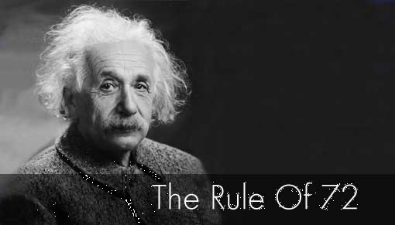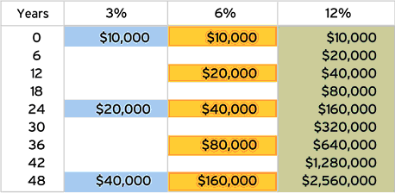Why know the "Rule of 72"?

Money rules apply to us
whether we know them or not.

Which interest rate would you rather have working for you?If we know the rule, and how it affects us, we can take advantage of the knowledge and make the money work for us instead of someone else!The Rule Of 72: Einstein’s Rate Of Return Formula
Albert Einstein created a fairly accurate “rule of thumb” mathematical formula for calculating how long it will take to double an investment based on the interest earned each year. That formula is commonly called the rule of 72. While it isn’t exact, it’s very close and can be a pretty accurate rule of thumb for quickly calculating how long it will take to double your money, based on it’s rate of return. Let’s take a look:

While he is noted for one formula (E=mc2) that became his trademark formula, it is quite complex and highly theoretical.

The formula he created for calculating how long it takes to double your money, on the other hand, is quite simple. He called this formula the rule of 72. When applied to a yearly rate of return (ROR), or annual percentage yield, you can quickly determine how long it will take to double your money. The rule of 72 formula looks like this:

72 / ROR = How long it will take to double your money.
For example, if you buy a \$1000 savings bond that earns 6% interest each year, you can apply the rule of 72 and discover that it will take 12 years for your original investment to double, or be worth \$2000. You can plug in the numbers and write it like this:

72 / 6% = 12 years
The rate of return is irrelevant to the formula. The rule always applies. Let’s look at another example. Let’s say you buy an indexed annuity that averages a yearly yield of 8%. Here’s how this example looks:

72 / 8.0% = 9.0 years
In the example above, it takes 9 years to double your money at a growth rate of 8%. So you can see that investing your money early and wisely is a good idea. It’s never too early to begin investing! The earlier, the better of course, but for anyone at any age, the time to start investing is now.

If you begin investing when you are say, 21 years old, you have more time to let your investment grow throughout your life than you would if you are say, 50 years old. This author believes that it really does not matter what age you are, whether you are 18 or 80, investing is a good thing. If not for you, the for your legacy…for your children and grandchildren. Think of the head start you can give them….

Compound interest is a truly marvelous thing for every investor to take advantage of. The rule of 72 is not an exact formula, just a rule of thumb, but it’s pretty close at any rate of return.

Do you know what Albert Einstein called the 8th wonder of the world? The answer is of course, compound interest.